# Examples of electrolysis with different substances

## Example 1: NaCl and waterSo what is happening at the electrodes?

Starting with the basic elements we have:-

### Hydrogen and oxygen### Sodium and chlorine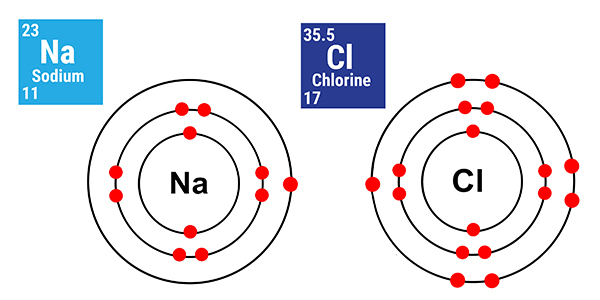### As molecules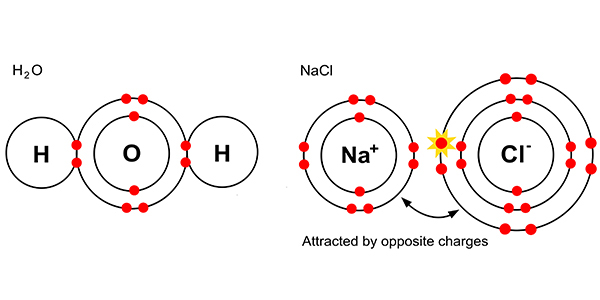But dissolved in solution with each other, chemists say we get:-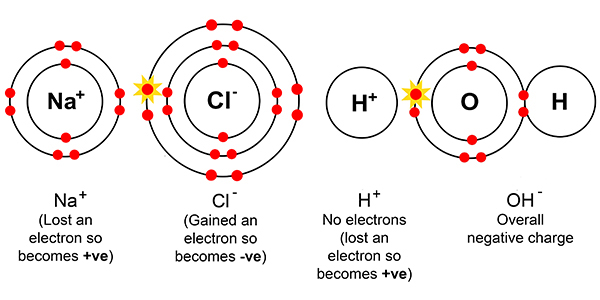### At the cathode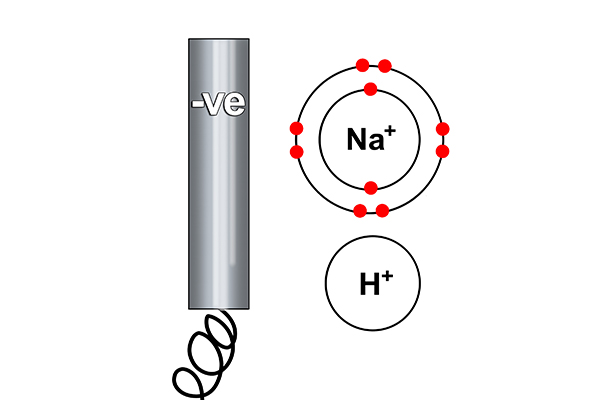Rule 1 says hydrogen forms at the cathode not sodium (as sodium is more reactive than hydrogen). So at the cathode electrons are given out (to the hydrogen ions).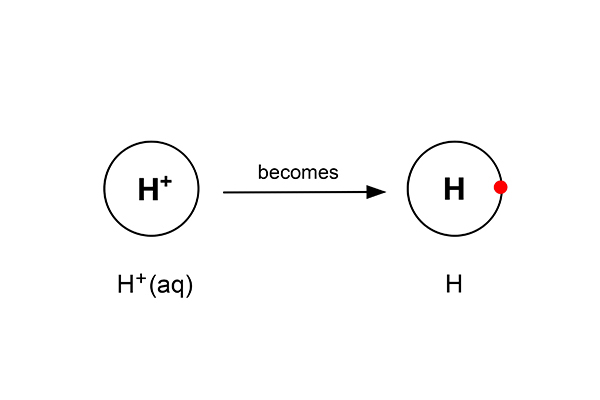But as hydrogen always goes around in pairs (as in a diatomic gas), two hydrogen atoms now combine to form a hydrogen gas.#### The half equation is:

H+ (aq)      +          ?          →        H2 (g)

To balance this                        2H+ (aq)     +          ?          →        H2 (g)

And the electron balance      2H+ (aq)     +          2e-          →        H2 (g)

Or                                                2H+ (aq)                             →        H2 (g) – 2e-

### At the anodeRule 2 says a halogen (Cl) forms at the anode and not a hydroxide (OH-).

So at the anode electrons are taken away from the chlorine.But as chlorine always goes around in pairs (as in a diatomic gas), two chlorine atoms now combine to form chlorine gas …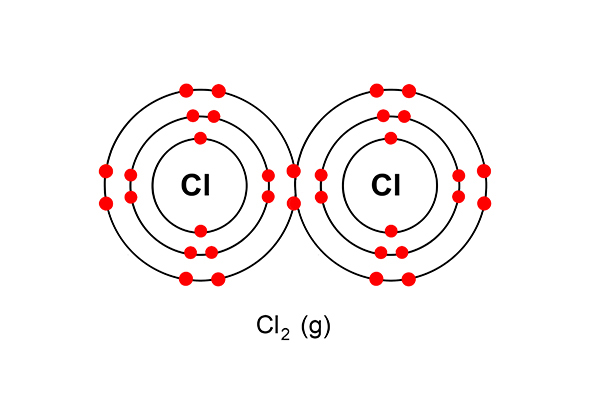#### The half equation is:

Cl- (aq)             +          ?          →        Cl2 (g)

To balance this                    2Cl- (aq)           +          ?          →        Cl2 (g)

And the electron balance   2Cl- (aq)           +          2e-         →        Cl2 (g)

Or                                            2Cl-                                           →        Cl2 + 2e-

The sodium and hydroxide ions just combine: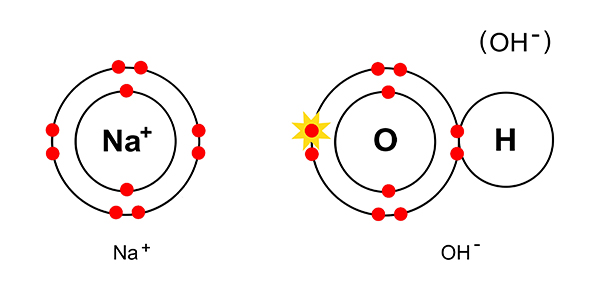They combine to give NaOH or (Na+OH-)NOTE: Within NaOH there is an ionic bond between sodium and oxygen and a covalent bond between oxygen and hydrogen.

This is a good example of how there can be different types of bond within one compound.